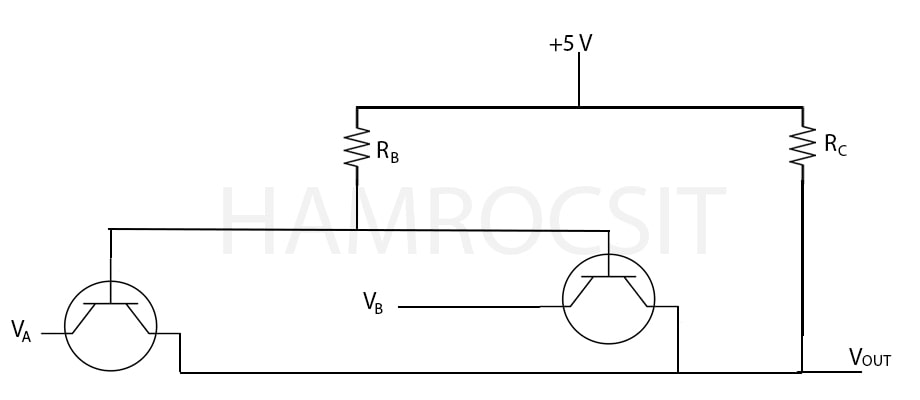Tribhuvan University

Institute of Science and Technology

2078

Bachelor Level / first-semester / Science

Computer Science and Information Technology( PHY113 )

Physics

Full Marks: 60 + 20 + 20

Pass Marks: 24 + 8 + 8

Time: 3 Hours

Candidates are required to give their answers in their own words as far as practicable.

The figures in the margin indicate full marks.

Group A

Attempt any TWO questions

1

Design electrical conductivity of semiconductors. Derive expression for conductivity in terms of impurity ionization energy. Give a plot to discuss “Theoretical temperature dependence of the electrical conductivity of an impurity semiconductor”.

2

Describe processes involved in the fabrication of integrated circuits include epitaxial growth, oxidation, oxide removal and pattern definition, doping (impurities in the Si), and interconnection of components.

3

Find expression for force on a current-carrying wire in a magnetic field to find the force experienced by a single charge.

Group B

Attempts any EIGHT Questions

4

Give spectrum of Hydrogen atom and discuss its lines.

5

Describe a brief account of Kronig-Penny model.

6

Describe the term “space quantization”.

7

A given spring stretches 0.1m when a force of 20N pulls on it. A 2-kg block attached to it on  a frictionless surface is pulled to the right 0.2m and released.

1. What is the frequency of oscillation of the block?
2. What are the velocity and acceleration when x = 0.12m, on the block’s first passing this point?
8

A proton is moving with a velocity v = (3 x 105 i + 7 x 105 k) m/sec in a region where there is a magnetic field B = 0.4j T. Find the force experienced by the proton.

9

A neutron spectroscopy a beam of mono energetic neutrons is obtained by reflecting reactor neutrons from a beryllium crystal. If separation between the atomic planes of the beryllium crystal is 0.732 A°, what is the angle between the incident neutron beam and the atomic planes that will yield a monochromatic beam of neutrons of wavelength 0.1 A°.

10
1. How many atomic states are there in hydrogen with n=3?
2. How are they distributed among the subshells? Label each state with the appropriates set of quantum numbers n, 1,m,m
3. Show that the number of states in a shell, that is ,states having the same n. is given by $$2n^2$$.
11

The density of aluminum is 2.70 g/cm3 and its molecular weight is 26.98 g/mole.

1. Calculate the Fermi energy
2. If the experimental value of EF is 12 eV, What is the electron effective mass in aluminum? [Aluminum is trivalent]
12

Analyze the circuit in the figure below. Determine the logic function performed by the circuit by making and justifying the appropriate truth table